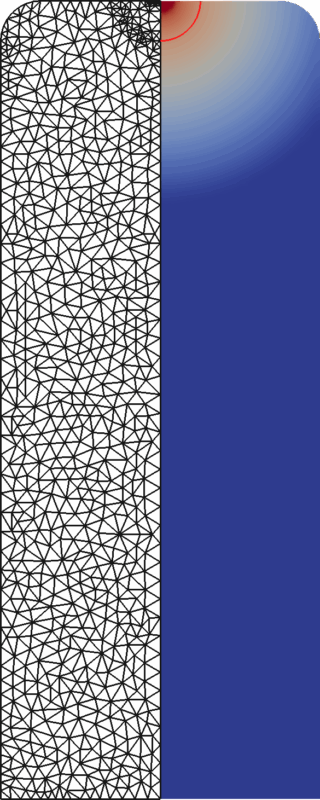# Solid-liquid phase transitions with a free melt surface

## Participants

### AM3

• Eberhard Bänsch
• Jordi Paul

• A. Schmidt
• T. Moshagen

## Aims of the projectMathematically speaking, we have the Navier-Stokes equations modelling the fluid flow in the liquid phase and the Stefan problem describing the heat transport and phase transformation. Both sets of equations are coupled through the convective heat transfer and by the geometry, as the phase transformation enlarges the liquid subdomain and the Navier-Stokes equations determine the position of the free capillary surface.

This nonlinear coupling is the mathematical challenge, as two overlapping moving boundaries governed by two different sets of equations have to be treated. The widely used enthalpy formulation of the Stefan problem does not track the movement of the phase boundary explicitly, which is needed for the flow simulation. Our approach is an Arbitrary Lagrangian Eulerian Finite Element method, which determines the phase boundary’s movement directly from the heat transport and incorporates this into the mesh movement. The mesh deformations can be rather large, so remeshing has to be done in addition to mesh moving, smoothing and equilibration techniques. All simulations are done with the 2d rotational symmetric version of our finite element code NAVIER.

Further information: Project Homepage at SFB 747, Bremen